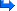TastySouP #1157 S6 Grievous Wounds Bug(1) Category: Bug ReportsJanuary-13-2016 9:22 AM PST (4 years ago)I was testing the damage modifiers on shadows 6 piece and I came across a bug.Impale - Grievous Wounds applies an extra 330% CHD on crit, but when this rune is used with Shadow's 6 Piece bonus it does not apply the extra CHD. The bonus is still active while using 2 or 4 pieces but becomes inactive with 6.Video demonstration for calculations:https://youtu.be/6c0iYmcwtkQNo Shadows:Dext: 315CHD:50Weapon Damage:34Non Crit in video = 1,033https://youtu.be/6c0iYmcwtkQ?t=40s=(skill damage*0.01) * weapon damage * (1+dext*0.01)=(750*0.01) * 34 * (1+305*0.01)=7.5 * 34 * 4.05=1,032.75Crit without grievous wounds in video = 1,549https://youtu.be/6c0iYmcwtkQ?t=44s=(skill damage*0.01) * weapon damage * (1+dext*0.01) * (1+(CHD+330)*0.01)=(750*0.01) * 34 * (1+305*0.01) * (1+50*0.01)=7.5 * 34 * 4.05 * 1.5=1,549.125Crit with grievous wounds in video = 4,957https://youtu.be/6c0iYmcwtkQ?t=1m4s=(skill damage*0.01) * weapon damage * (1+dext*0.01) * (1+(CHD+330)*0.01)=(750*0.01) * 34 * (1+305*0.01) * (1+(50+330)*0.01)=7.5 * 34 * 4.05 * 4.8=4,957.2Shadows 2PC:Dext: 1627CHD:99%Weapon Damage:34Shadows 2pc:x1000%Non Crit in video = 48,442https://youtu.be/6c0iYmcwtkQ?t=1m39s=(skill damage*0.01) * weapon damage * (1+dext*0.01) * (1+S2*0.01)=(750*0.01) * 34 * (1+1627*0.01) * (1+1000*0.01)=7.5 * 34 * 17.27 * 11=48,442.35Crit without grievous wounds in video = 94,600https://youtu.be/6c0iYmcwtkQ?t=1m40s=(skill damage*0.01) * weapon damage * (1+dext*0.01) * (1+CHD*0.01) * (1+S2*0.01)=(750*0.01) * 34 * (1+1627*0.01) * (1+99*0.01) * (1+1000*0.01)=7.5 * 34 * 17.27 * 1.99 * 11=96,400.2765Crit with grievous wounds in video = 256,260https://youtu.be/6c0iYmcwtkQ?t=1m59s=(skill damage*0.01) * weapon damage * (1+dext*0.01) * (1+(CHD+330)*0.01) * (1+S2*0.01)=(750*0.01) * 34 * (1+1627*0.01) * (1+(99+330)*0.01) * (1+1000*0.01)=7.5 * 34 * 17.27 * 5.29 * 11=256,260.0315Shadows 4PC:Dext: 3461CHD:99%Weapon Damage:34Shadows 2pc:x1000%Non Crit in video = 99,886https://youtu.be/6c0iYmcwtkQ?t=2m35s=(skill damage*0.01) * weapon damage * (1+dext*0.01) * (1+S2*0.01)=(750*0.01) * 34 * (1+3461*0.01) * (1+1000*0.01)=7.5 * 34 * 35.61 * 11=99,886.05Crit without grievous wounds in video = 198,773https://youtu.be/6c0iYmcwtkQ?t=2m34s=(skill damage*0.01) * weapon damage * (1+dext*0.01) * (1+CHD*0.01) * (1+S2*0.01)=(750*0.01) * 34 * (1+3461*0.01) * (1+99*0.01) * (1+1000*0.01)=7.5 * 34 * 35.61 * 1.99 * 11=198,773.2395Crit with grievous wounds = https://youtu.be/6c0iYmcwtkQ?t=3m=(skill damage*0.01) * weapon damage * (1+dext*0.01) * (1+(CHD+330)*0.01) * (1+S2*0.01)=(750*0.01) * 34 * (1+3461*0.01) * (1+(99+330)*0.01) * (1+1000*0.01)=7.5 * 34 * 35.61 * 5.29 * 11=528,397.2045Shadows 6PC:Dext: 4496CHD:99%Weapon Damage:34Shadows 2pc:x1000%Shadows 6pc:+40000%Non Crit = 7,004,534https://youtu.be/6c0iYmcwtkQ?t=3m40s=((skill damage+Shadows 6pc)*0.01) * weapon damage * (1+dext*0.01) * (1+S2*0.01)=((750+40000)*0.01) * 34 * (1+4496*0.01) * (1+1000*0.01)=407.5 * 34 * 45.96 * 11=7,004,533.8Crit without Grievous Wounds = 13,939,022https://youtu.be/6c0iYmcwtkQ?t=3m39s=((skill damage+Shadows 6pc)*0.01) * weapon damage * (1+dext*0.01) * (1+CHD*0.01) * (1+S2*0.01)=((750+40000)*0.01) * 34 * (1+4496*0.01) * (1+99*0.01) * (1+1000*0.01)=407.5 * 34 * 45.96 * 1.99 * 11=13,939,022.262Crit with grievous wounds in game = 13,939,022 ? bugged, missing CHDhttps://youtu.be/6c0iYmcwtkQ?t=3m39s → select Grievous Woundshttps://youtu.be/6c0iYmcwtkQ?t=4m2s → crit with Grievous Wounds=((skill damage+Shadows 6pc)*0.01) * weapon damage * (1+dext*0.01) * (1+(CHD+330)*0.01) * (1+S2*0.01)=((750+40000)*0.01) * 34 * (1+4496*0.01) * (1+(99+330)*0.01) * (1+1000*0.01)=407.5 * 34 * 45.96 * 5.29 * 11=37,053,983.802Here's another Shadow's 6 piece bug:http://us.battle.net/d3/en/forum/topic/20419192810Nevalistis S6 Grievous Wounds Bug(3) January-21-2016 4:39 PM PST (4 years ago)Grievous Wounds not affecting the 6-piece bonus of Shadow's Mantle is not a bug. In fact, none of the damage bonuses from runes for Impale affect the 6-piece bonus. This is to preserve diversity rather than leave one rune as the obvious, mathematically correct choice. Players shouldn't feel punished because of their set bonus if they'd rather use another rune or element. On the flip side, the other issue you mention with Convention of Elements does appear to be a bug. The 6-piece bonus of Shadow's Mantle is intended to deal the elemental type of whatever Impale rune is selected, thereby benefitting from Convention of Elements when the appropriate element is active. We've put in a report for this and will be investigating it.